# Bayesian model fitting

Last time we fit the average behavior of people by finding the value of sigma that minimizes the sum of the squared errors - the "least squares" solution. This is a good first step for model fitting, but the choice of minimizing the square of the error is somewhat arbitrary. We can do better by taking a more principled approach to model fitting based on Bayesian inference.
Note: Today's class will have more Math than usual. Don't worry if you feel it's going over your head, the actual code is no more difficult than anything we've done before.

## Inferring sigma using Bayesian inference

Before we start let's revisit the error vs sigma curve we plotted last week.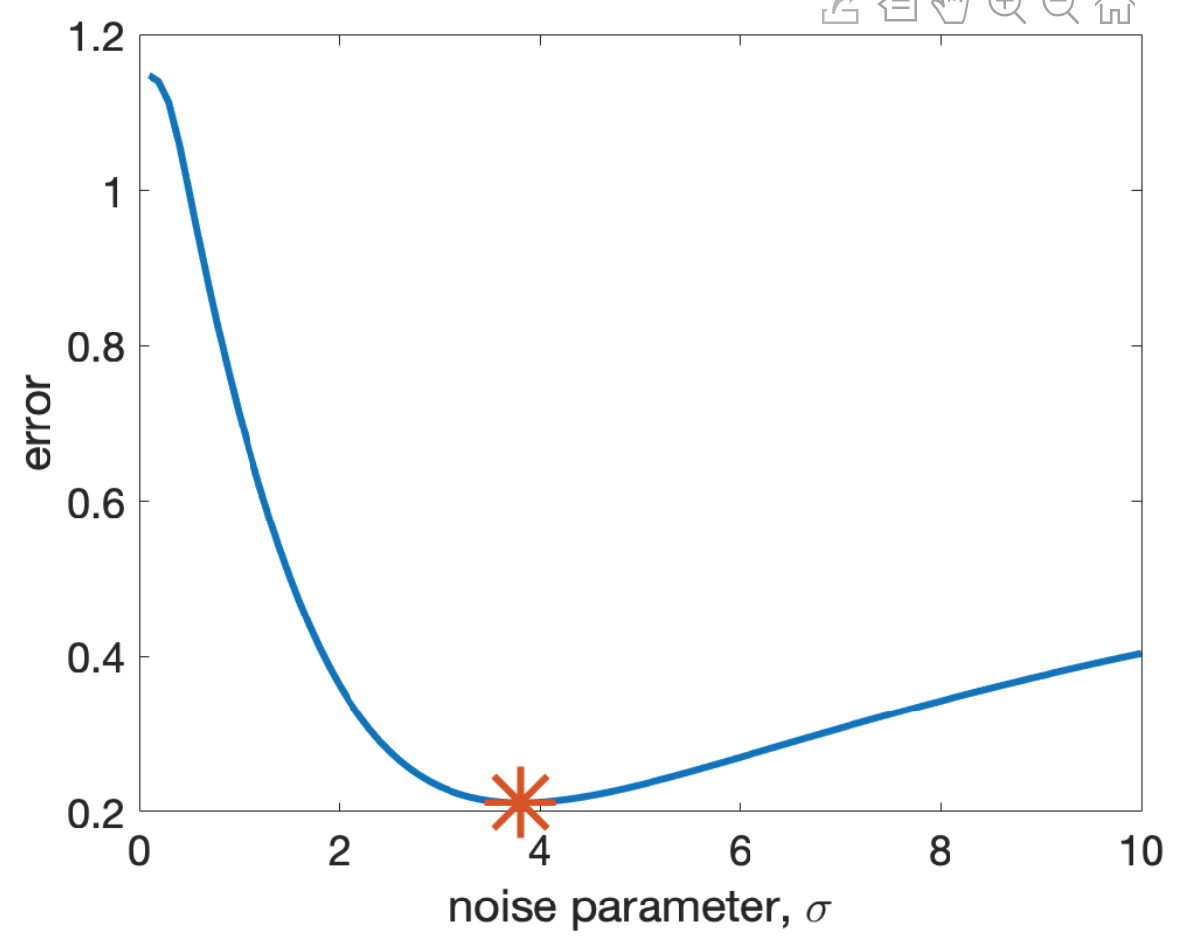Here we had a minimum error at sigma = 3.812 and we took that as our best fitting value of sigma. But what are the error bars on that best fitting value? If I change sigma to 3.7 how much worse do I do? What about sigma = 3? or sigma = 4? From the plot it's clear that changing sigma by a small amount around 3.812 isn't going to change the error by much. What I'd like to do is be able to say something about the range of sigma values that give good fits.
One way to get a handle on this range is to compute, not a best fitting value of sigma, but a probability distribution over what the best fitting value of sigma would be. So ideally we would want something like this, where the peak of the distribution is at 3.812, but it's also possible that the best fitting value of sigma is 4 or even 5.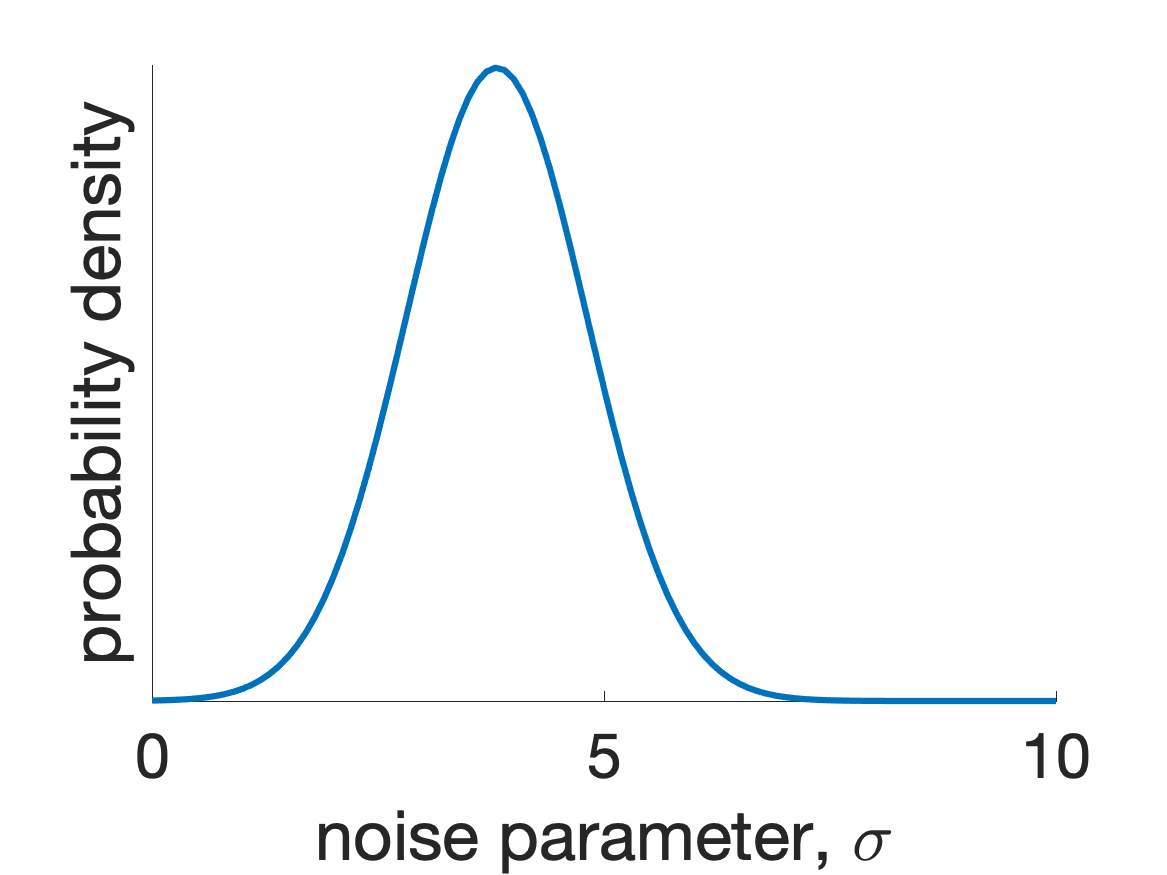In this way a probability distribution quantifies our uncertainty over sigma.

### Computing the posterior distribution over sigma given the data

It turns out that we can compute the probability over sigma using Bayes Rule. If you are not familiar with Bayes Rule don't worry too much, it's just an equation that tells you the relationship between different types of probabilities. I'm going to build it up with reference to our specific example of fitting sigma.
First, we have to consider the distribution we want to derive. What we'd like to compute is the probability distribution over sigma given the behavioral data from our experiment. In Mathematics this is called a conditional distribution and it's written like this ...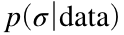where the p means probability, the first argument, σ means that's the thing we want the probability distribution to be over, the can be read as "given" or "conditioned on" and the data is all the information from our experiment (the expected values of each question and the choices of the participants).
So we can read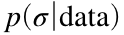as "the probability distribution over sigma given the data."
Another name foris the posterior distribution because this is the distribution we compute after we incorporate the data. Our goal is to compute the posterior, but we can't do it yet. Our model doesn't tell us anything about the posterior - at least not directly. Instead, our model gives us information about the likelihood ...

### The likelihood

While our goal is the posterior, almost by definition, our model gives us another probability distribution known as the likelihood. In this context, the likelihood tells us about the probability of observing a given set of data given a particular value of sigma. In Math notation this is the "reverse" of the posterior ...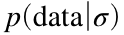How does our model specify this probability distribution? Well, first let's consider what the data points actually are. In our experiment the most important part of the data are the choices that people made (which our model assumes are based on the expected values we controlled in the experiment). So we can write the data as just a list of choices, like this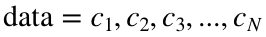where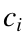is the choice on question i and N is the total number of questions we are considering. To shorten how we write this list we often write the data like this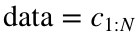where the 1:N part is a bit like Matlab notation saying that we want the choices from choice 1 to choice N.
Now, for any particular choice, say choice i, our model actually defines the probability distribution over that choice for a given value of sigma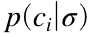- can you think what that might be?
It's the softmax function itself! That is, by the definition of our model, the probability that people choose the safe option on trial i iswhereConversely, the probability that they choose the risky version is justNow, if we assume that each choice is completely independent of the other choices, then we can use these expressions to compute the probability over all N choices as just the product of the probabilities of the N individual choices like this ...where the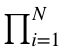symbol means "take the product over all terms with i going from 1 to N."
Now we have something we can actually compute ...

### Computing the likelihood in Matlab

Let's make things more concrete by computing the likelihood for a particular value of sigma = 5using our data set. First load the data
clear
% compute expected values
EV_safe = 10;
EV_risky = P.*V;
% set value of sigma
sigma = 5;
% also compute beta to make softmax equation easier
beta = 1 /sigma * sqrt(pi/2);
Then let's compute a likelihood of just a single choice - the first choice of the first person,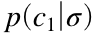. Now, this very much depends on what the person chose. If they chose the safe option thenBut if they chose the risky option, the likelihood of the first choice isSo we are going to need an if statement to compute the probability depending on the choice ...
if rsk(1,1) == 0
% chose safe option
p_cGivenSigma = 1 / (1 + exp( beta * (EV_risky(1) - EV_safe)));
else
% chose risky option
p_cGivenSigma = 1 - 1 / (1 + exp( beta * (EV_risky(1) - EV_safe)));
end
p_cGivenSigma
p_cGivenSigma = 0.5000
Note: we only use EV_risky(1) because we want to know about the likelihood of just the first question
Great! So we've computed the likelihood of one choice. Now let's repeat the process for all choices and all subjects. To do this I'm going to need two loops, one for participants and one for questions ...
for i = 1:size(rsk, 1) % loop over participants
for j = 1:size(rsk, 2) % loop over questions
if rsk(i,j) == 0
% chose safe option
p_cGivenSigma(i,j) = 1 / (1 + exp( beta * (EV_risky(j) - EV_safe)));
else
% chose risky option
p_cGivenSigma(i,j) = 1 - 1 / (1 + exp( beta * (EV_risky(j) - EV_safe)));
end
end
end
p_cGivenSigma
p_cGivenSigma = 146×12
0.5000 0.2193 0.2690 0.3160 0.3784 0.4336 0.4966 0.5560 0.6219 0.6760 0.7248 0.7769 0.5000 0.7807 0.7310 0.6840 0.6216 0.5664 0.5034 0.4440 0.6219 0.6760 0.7248 0.7769 0.5000 0.2193 0.2690 0.3160 0.3784 0.4336 0.5034 0.5560 0.6219 0.3240 0.2752 0.7769 0.5000 0.7807 0.7310 0.6840 0.6216 0.5664 0.5034 0.5560 0.6219 0.3240 0.2752 0.7769 0.5000 0.7807 0.7310 0.6840 0.6216 0.5664 0.4966 0.4440 0.6219 0.6760 0.7248 0.7769 0.5000 0.7807 0.7310 0.6840 0.6216 0.5664 0.5034 0.5560 0.6219 0.3240 0.7248 0.7769 0.5000 0.7807 0.7310 0.3160 0.6216 0.4336 0.5034 0.5560 0.6219 0.6760 0.2752 0.7769 0.5000 0.7807 0.7310 0.6840 0.6216 0.5664 0.5034 0.4440 0.6219 0.6760 0.7248 0.7769 0.5000 0.7807 0.7310 0.6840 0.6216 0.5664 0.4966 0.5560 0.6219 0.6760 0.7248 0.7769 0.5000 0.7807 0.7310 0.6840 0.6216 0.4336 0.4966 0.4440 0.6219 0.3240 0.2752 0.7769
Note a few things here:
• I'm storing the likelihoods of each trial in a matrix p_cGivenSigma(i,j)
• I'm using the size function to get the relevant dimensions of rsk
• size(rsk,1) gets the size of the first dimension of rsk - i.e. the number of participants (146 for me, larger for your complete data set)
• size(rsk,2) gets the size of the second dimension of rsk - i.e. the number of questions (12)
Now I've got a matrix telling me the probabilities of every choice made by all participants, I just need to take the product of all of these probabilities to get the overall likelihood. I can do this using the "prod" command in Matlab. I'll need to use it twice though, once to get the product over the rows and a second time to get the product over the columns ...
likelihood = prod(prod(p_cGivenSigma))
likelihood = 0
Hmmmmm .... so my likelihood - i.e. the probability of making all these choices - is zero? That doesn't seem right and, in fact, it is not right. Matlab has made a math error!
What's happened is that Matlab has automatically rounded the likelihood down to zero. Why? Because of how computers represent numbers. In particular, a computer can only represent a number to a particular level of precision. To see this, copy and paste this number into the Command Line
0.00000000000000000000000000000000000000000000000000000000000000000000000000000000000000000000000000000000000000000000000000000000000000000000000000000000000000000000000000000000000000000000000000000000000000000000000000000000000000000000000000000000000000000000000000000000000000000000000000000000000000000000000000000000000000000000000000000000000000000000000000000000000000000000000000000000000000000000000000000000000000000000000000000000000000000001
0.00000000000000000000000000000000000000000000000000000000000000000000000000000000000000000000000000000000000000000000000000000000000000000000000000000000000000000000000000000000000000000000000000000000000000000000000000000000000000000000000000000000000000000000000000000000000000000000000000000000000000000000000000000000000000000000000000000000000000000000000000000000000000000000000000000000000000000000000000000000000000000000000000000000000000000001
ans = 0
Matlab returns zero - it simply doesn't have the ability to represent a number to this many decimal places.
It turns out that our likelihood is similarly small. This is because the likelihood is a product of small numbers. By definition each probability is a small number (between 0 and 1) and if I take the product of a bunch of small numbers I get an even smaller number. In my data set I have 1752 choices (12 choices for each of 146 participants) and so I'm taking the product of 1752 small numbers, which is a very small number indeed.
To get around this problem I need to take logs ...

### Computing the log likelihood in Matlab

Even though Matlab can't deal with very small numbers directly, it can deal with the logarithm of very small numbers. This is because the logarithm of a very small number is negative number with a large magnitude. For example the 0.0000 ... 1 example above has 451 zeros, so it's equal to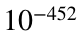. If I take the log of this (in base 10) I get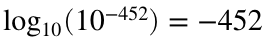a much more managable number. Moreover, if you remember from your Math classes, taking the log of a product is the same as taking the sum of the logs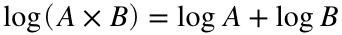and soSo, to compute the log likelihood I just need to compute the sum of the logs of the individual choice probabilities (Note: I need to use sum twice to get the sum over both dimensions of the matrix).
logLikelihood = sum(sum(log10(p_cGivenSigma)))
logLikelihood = -452.0762
So the likelihood is about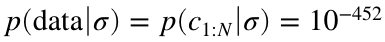- i.e. almost exactly the same size as that very small number we wrote out above.

### From likelihood to posterior ...

OK so we can compute the log probability of any data set for a given value of sigma - that's the log likelihood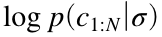. But what we want is the posterior, the probability distribution over sigma given the data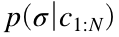.
How do we go from likelihood to posterior? That's where the magic of Bayes Rule comes in. In particular, Bayes rule tells us that the posterior and the likelihood should be related like this ...where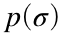is the prior distribution on σ - i.e. what values we think sigma could be before we consider the data - and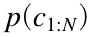is the prior probability of the data averaging over all values of sigma.
The prior distribution,, codes what we know about σ before we do the experiment. In most cases this isn't very much. In our case we only know that σ must be bigger than zero. A simple assumption is to just say it's a constant for positive σ (there are other assumptions you can make, but for our purposes a constant is fine).
The prior probability of the datais a trickier term to get your head around, but for our purposes we can think of this term as a normalizing constant, i.e. a constant that we divide by to ensure that the posterior distribution is an actual probability distribution in that its integral adds up to one, i.e. thatThis we can ensure by writing### Computing the posterior in Matlab

In Matlab we can actually compute the posterior in this case. But first we need to do something about the logs. Specifically, we have the log likelihood,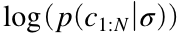, but Bayes Rule needs the raw likelihood,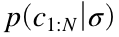. However, we can't just compute this raw likelihood by taking the exponential of the log likelihood like this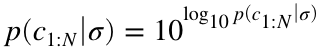because the number we get is so small that Matlab rounds it to zero
lik = 10^logLikelihood
lik = 0
How can we get around this? Well let's first imagine computing the exponential of something related to the log likelihood but not exactly the same. In particular, let's take the exponential of the log liklihood plus some constant number k and let's call the result of this X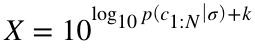Now, if k is big enough (say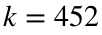for now) then Matlab will be able to compute X correctly ...
k = 452;
X = 10^(logLikelihood + k)
X = 0.8390
Now, the key thing to realize here is that X is related to the true likelihood by a factor of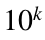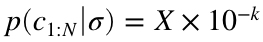If we substitute this expression into Bayes rule, we get ...But note that the fraction on the right has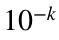on the top and the bottom so those terms cancel! In addition, we assumed thatwas a constant so those terms cancel too and we are left withSo, we can compute X to finally get to the posterior we just need to compute the integral of X over all values of sigma.

### The integral of X over σ

An integral is really just a sum, so we can approximate the integral of X over σ by computing X for a bunch of sigma values and then adding them all up. We can do this using a loop over sigma values.
First let's make a function to compute the log-likelihood for any sigma value ...
function logLikelihood = computeLogLikelihood(EV_safe, EV_risky, rsk, sigma)
beta = 1 /sigma * sqrt(pi/2);
for i = 1:size(rsk, 1) % loop over participants
for j = 1:size(rsk, 2) % loop over questions
if rsk(i,j) == 0
% chose safe option
p_cGivenSigma(i,j) = 1 / (1 + exp( beta * (EV_risky(j) - EV_safe)));
else
% chose risky option
p_cGivenSigma(i,j) = 1 - 1 / (1 + exp( beta * (EV_risky(j) - EV_safe)));
end
end
end
logLikelihood = sum(sum(log10(p_cGivenSigma)));
Then let's do the loop to compute the log-likelihood for a bunch of sigma values
sigma_vals = [0.01:0.01:10];
for i = 1:length(sigma_vals)
logLikelihood(i) = computeLogLikelihood(EV_safe, EV_risky, rsk, sigma_vals(i));
end
Let's plot that log-likelihood just to see what it looks like ...
clf;
plot(sigma_vals, logLikelihood, 'LineWidth', 3)
xlabel('noise parameter, \sigma')
ylabel('log likelihood')
set(gca, 'fontsize', 18)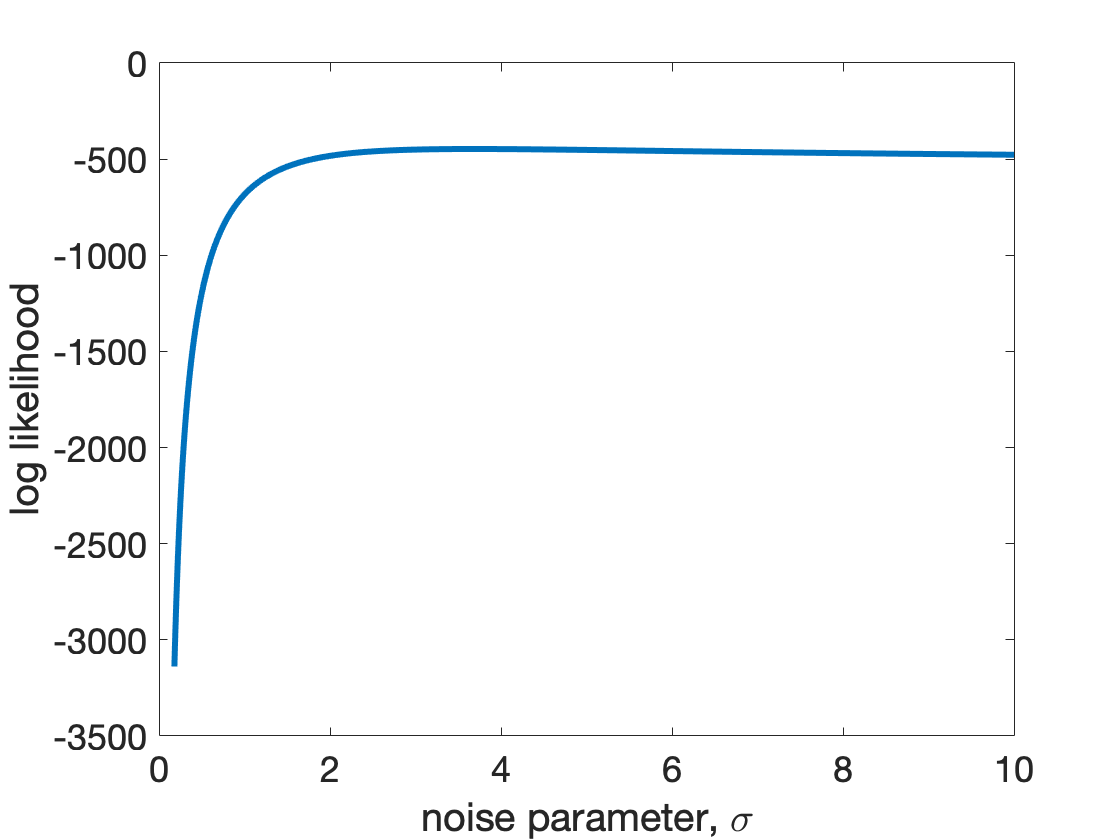Note that this has a peak around sigma = 4 - we'll find out exactly where later.
Now let's set k to be minus the maximum value of the log likelihood. This ensures that the biggest value of X will be 1.
k = -max(logLikelihood);
X = 10.^(logLikelihood + k);
Let's plot X too ...
clf
plot(sigma_vals, X, 'linewidth', 3)
xlabel('noise parameter, \sigma')
ylabel('X')
set(gca, 'fontsize', 18)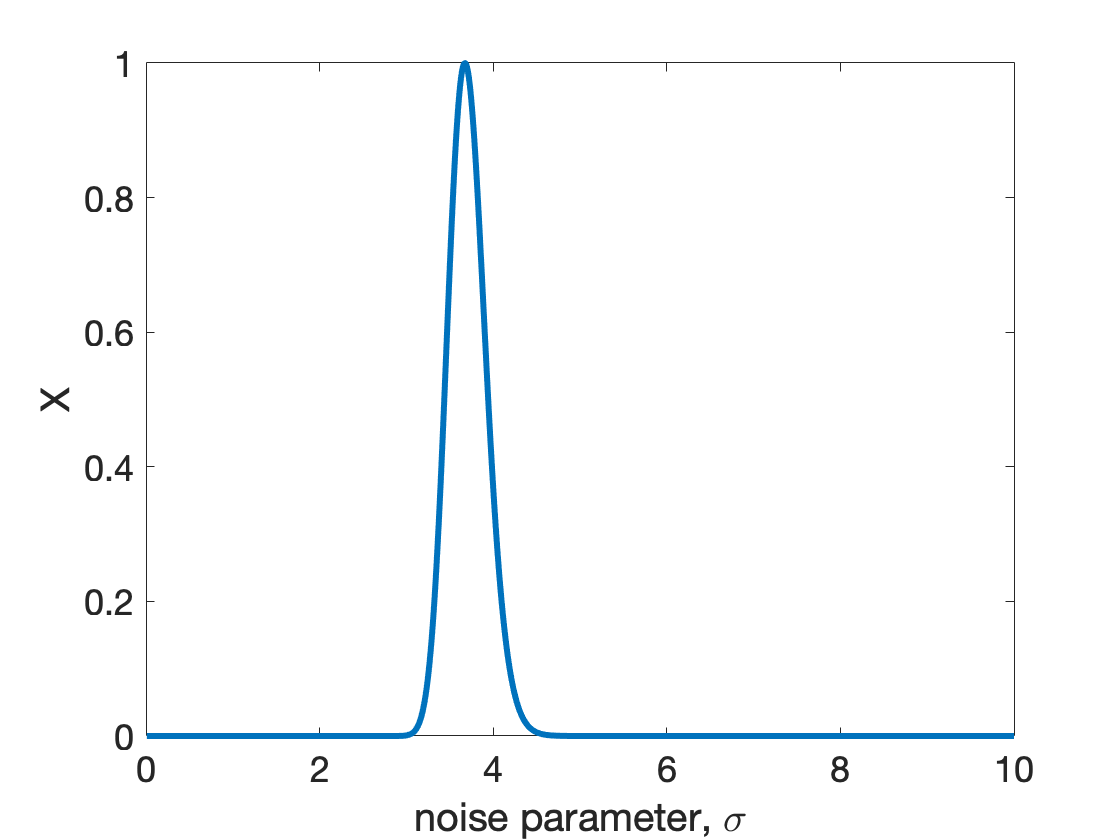This is starting to look like a probability distribution, but you can see that the integral of this distribution will be bigger than 1.
Next, let's compute (or at least approximate) the integral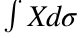integral = sum(X);
and then finally, we can compute the posterior by dividing X by the integral
posterior = X / integral;
clf;
plot(sigma_vals, posterior, 'LineWidth', 3)
xlabel('noise parameter, \sigma')
ylabel('posterior p(\sigma | data)')
set(gca, 'fontsize', 18)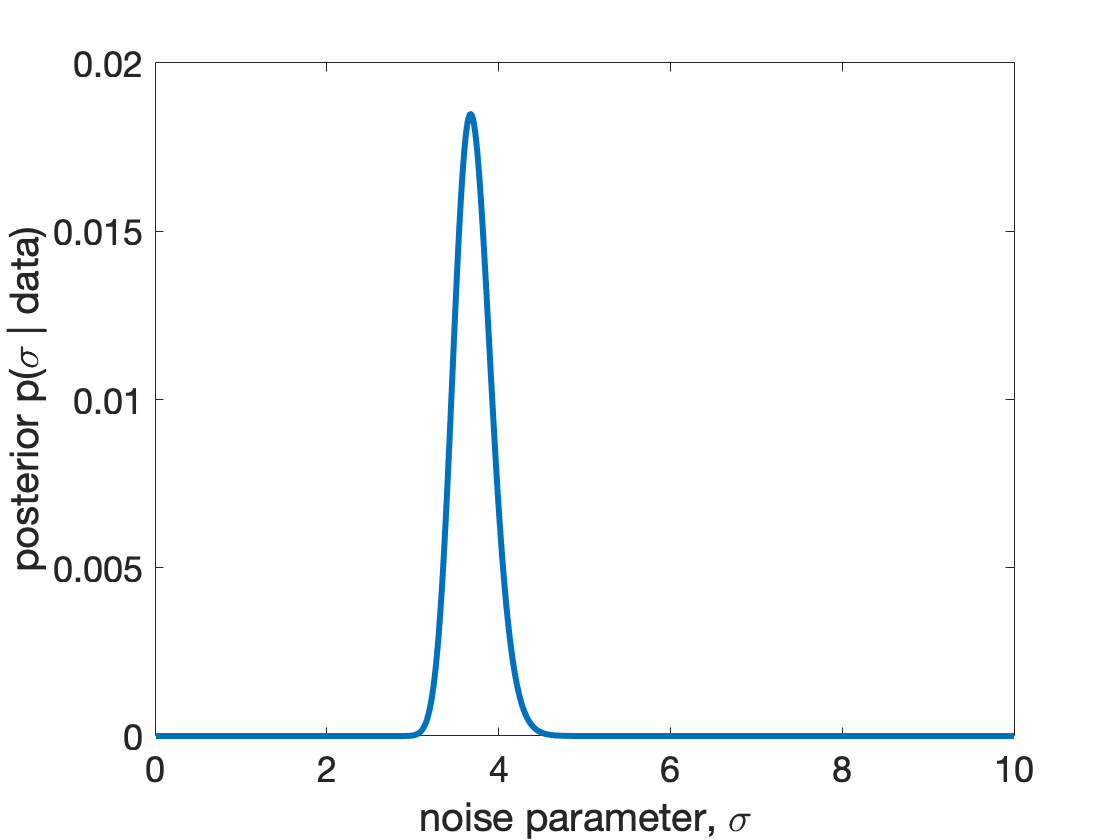Note that the shape of this distribution is exactly the same as the shape of X. That's because to get the posterior we simply scaled X by a constant.
This posterior tells us about our uncertainty in the value of sigma - the peak is still around 3.8, but values of 3.5 or 4 are totally possible, while values of 2 or 5 are highly unlikely.